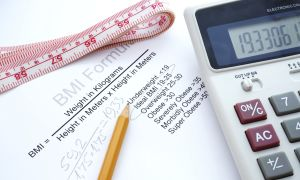How is lean body mass measured?Lean body mass is the weight of your body that doesn't come from fat. You'll commonly use your lean body mass to measure your progress in a weight training program. You can calculate it from specific body measurements which you can make at home. Separate formulas for men and women are used to determine lean body mass. According to professional bodybuilder and author Hugo Rivera's article at Dave Draper's Iron Online, these formulas are accurate to within +/- three percent of the real value.

Step 1

Weigh yourself in pounds with a scale. Measure your waist in inches at the level of your navel. Perform measurements when you first wake up so that your body weight will be at its lowest level of the day.

Step 2

Take additional measurements if you're female. Measure the circumference of one of your wrists, one forearm and your hips in inches at their fullest points.

Step 3

Calculate your lean body mass with the following equation if you're male: Lean Body Mass = (weight x 1.082) -- (waist x 4.15) + 94.42.

Let your waist measurement be 34 inches and let your weight be 179 pounds for this example. You have a lean body mass of (179 x 1.082) -- (34 x 4.15) + 94.42 = 147 lb.

Step 4

Compute your lean body mass with the following equation if you're female: Lean Body Mass = (weight x 0.732) -- (waist x 0.157) + (wrist / 3.14) + (0.434 x forearm) -- (0.249 x hips) + 8.987 = 111 lb.

Let your waist measurement be 32 inches and let your weight be 136 lb. for this example. Your wrist measurement is 6.5 inches, your forearm measurement is 10 inches and your hip measurement is 39 inches. You have a lean body mass of (136 x 0.732) -- (32 x 0.157) + (6.5 / 3.14) + (0.434 x 10) -- (0.249 x 39) + 8.987 = 103 lb.

Provided by Allan Robinson on Jul 27, 2010 at livestrong.comLean body mass is estimated using any of the various tests used to measure body composition.  Examples include skin fold calipers, hydrostatic weighing, Bod Pod, bioelectrical impedance, or DEXA.

Continue Learning about Body Mass Index (BMI)Is Your BMI a Lie?
Body mass index (BMI), a simple ratio of height and weight, has become a shorthand way for doctors and insurance companies to determine if someone is ...Dr. Mike Clark, DPTDr. Michael Roizen, MD